The following table shows the harmonics for the surface of a sphere, reduced to a hemisphere – up to “l=5”.

These functions are usually called Y(l,m) and are obtained from the
associated Legendre functions
P(l,m) by setting

Y(l,m) := P(l,m)(cos (theta)) * exp(i*m*phi)

where theta gives the angle with the equatorial plane, and phi the angle with respect to some fixed direction within that plane.

In the pictures, the values of the functions are colour coded: green for low,
blue for intermediate and red for high. See also the colour bar at the bottom
of each picture. In addition the colour bar also indicates the shade corresponding to the value 0 by a black line.

The left two pictures illustrate the imaginary part of these functions, and the right two pictures the real part. The first of each pair gives the view of from a 30 degree angle in respect to the equatorial plane, the second one from a 90 degree angle (the top).

More on surface spherical harmonics can be found in books on mathematical physics, for example Mathematical methods for physicists by George Arfken. Also they are important in quantum mechanics. See the Spherical Harmonics Gallery Page for a different representation.

l=1
m=0m=1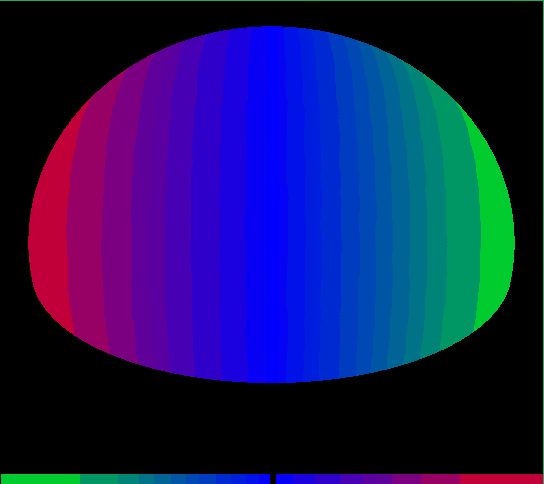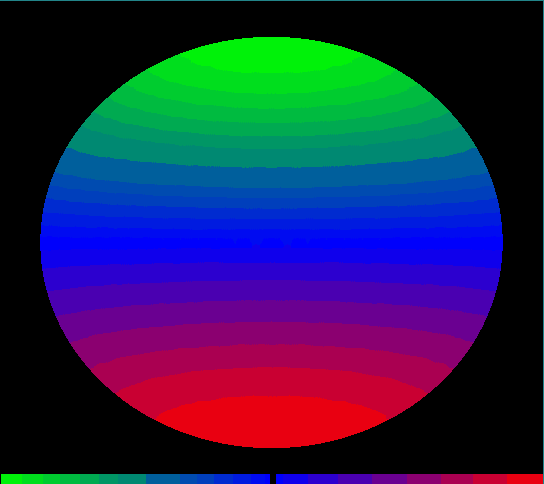l=2
m=0m=1m=2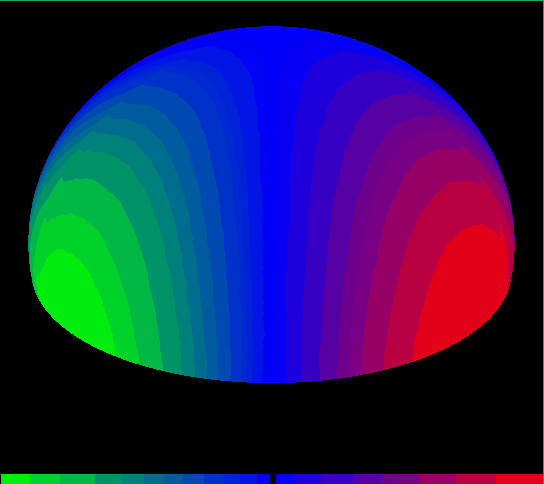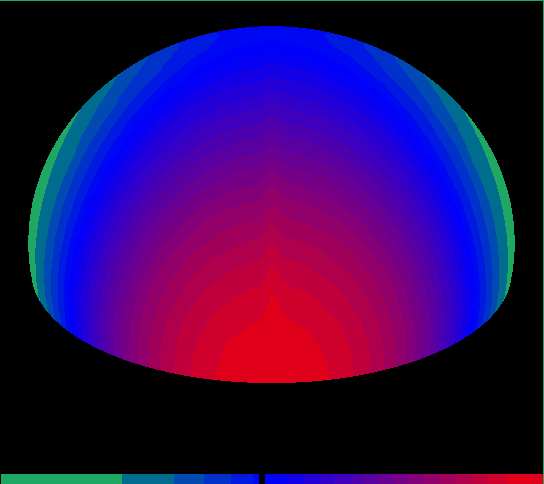l=3
m=0m=1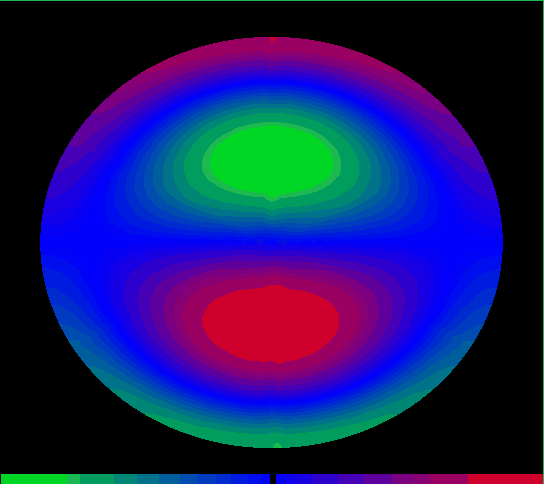m=2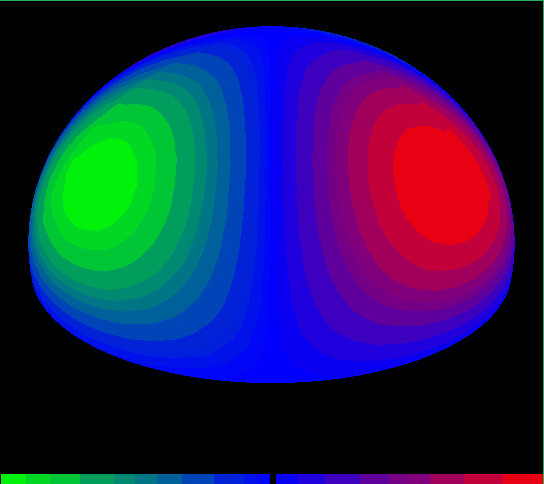m=3l=4
m=0m=1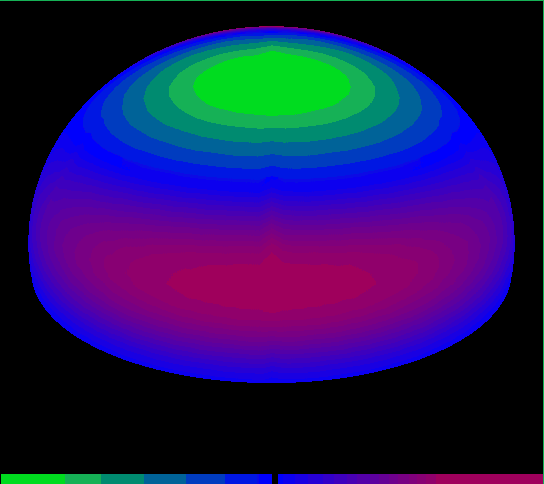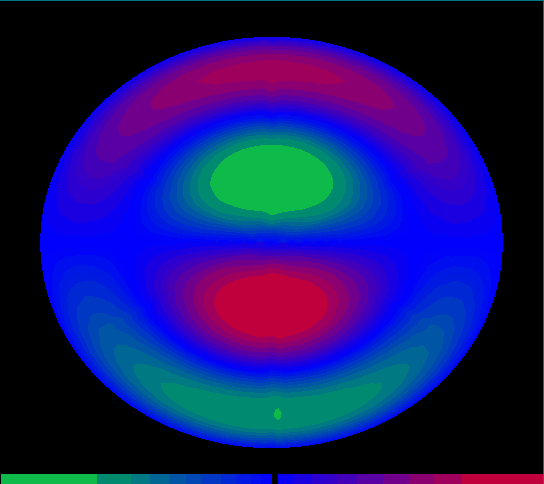m=2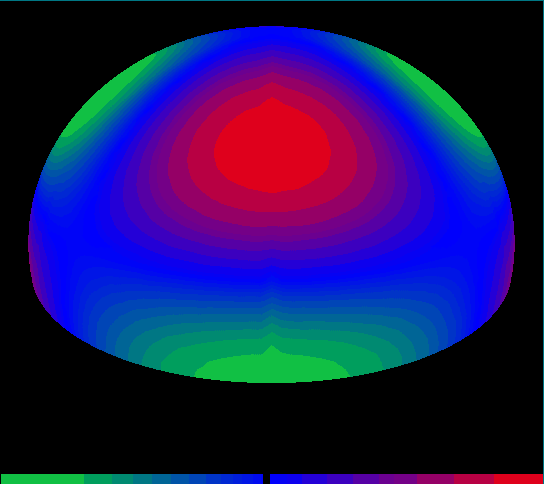m=3m=4l=5
m=0m=1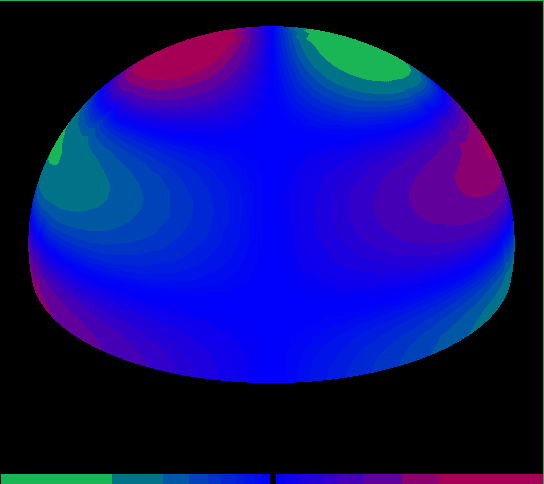m=2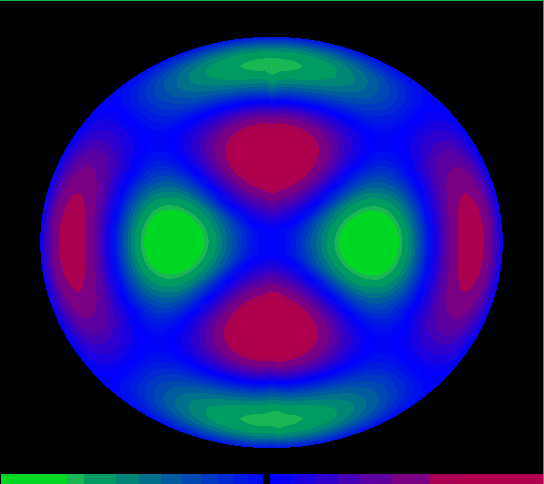m=3m=4m=5